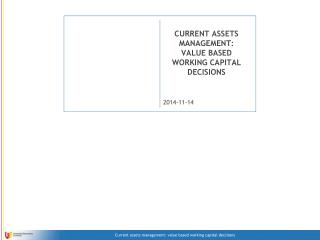DownloadDownload PresentationCURRENT ASSETS MANAGEMENT: VALUE BASED WORKING CAPITAL DECISIONS

# CURRENT ASSETS MANAGEMENT: VALUE BASED WORKING CAPITAL DECISIONS

Télécharger la présentation## CURRENT ASSETS MANAGEMENT: VALUE BASED WORKING CAPITAL DECISIONS

- - - - - - - - - - - - - - - - - - - - - - - - - - - E N D - - - - - - - - - - - - - - - - - - - - - - - - - - -
##### Presentation Transcript

1. CURRENT ASSETS MANAGEMENT: VALUE BASED WORKING CAPITAL DECISIONS • E-mail: GRZEGORZ.MICHALSKI@UE.WROC.PL • www: HTTP://MICHALSKIG.UE.WROC.PL/ • Mobile: 0503452860 • 5 lectures + 1 exam (test) • Next lecture: 20th October. • T. S. Maness, J. T. Zietlow, „Short-Term Financial Management”. • N. C. Hill, W. L. Sartoris, Short-term financial management: text and cases, Prentice Hall, 2004.

2. Basic financial aim of the firm • Firm value maximization: Where: FCFn = free cash flows, k = cost of capital financing the firm(WACC) n = period in which FCFn will be generated

3. FCF – how to calculate? k – cost of capital, how to value?

4. Cash cycle & Operating Cycle

5. Cash cycle & Operating Cycle • Operating cycle - the time period from commitment of cash for purchases until the collection of receivables resulting from the sale of goods/services. • Operating cycle = Inventory period + A/cs receivable period • Cash cycle - the time period from the actual outlay of cash for purchases until the collection of receivables. • Cash cycle = Operating cycle - A/cs payable period

6. Example: Cash cycle & Operating Cycle • The following information has been provided: • CR = 720 • Inventory = 60 • Accounts receivable = 80 • Accounts payable = 50 • Calculate OKZAP, DSO, OOSZwD, CO, CKG. • If we know that operating cash will be held on the level of 2 days sale, how much money will be tied in NWC (Net Working Capital)?

7. How changes in curent assets influence value? CS: Inventory period = 35 days, Accounts receivable period = 26 days, accounts payable period = 20 days, Cash Revenues = 1234, T=19%, calculate: • Assets, if FA = 800 • Capital Involved • FCF0, FCF1-n, FCFn • IRR • Cost of Capital if D/(D+E) = 40%, kd = 13% & ke = 34%. • NPV What will change if IP is shorter? Longer? What will change if ARP is shorter? Longer? What will change if APP is shorter? Longer?

8. Liquid assets level & Firm Value

9. Liquidity level & Profitability

10. Liquidity level & Value of Liquidity Where: vi = intrinsic (internal) value of liquidity, vm = market value of liquidity, pp1 = liquidity level (1) for vi > vm ppopt = optimal liquidity level for vi = vm

11. Liquidity level & Value of Liquidity Where: vi = intrinsic (internal) value of liquidity, vm = market value of liquidity, pp2 = liquidity level (2) for vi < vm ppopt = optimal liquidity level for vi = vm